xInfinitesimalOverview

Infinitesimals have been used to express the idea of objects so small that there is no way to see them or to measure them. The word infinitesimal comes from a 17th century Modern Latin coinage infinitesimus, which originally referred to the "infinite-th
Ordinal number (linguistics)
In linguistics, ordinal numbers are the words representing the rank of a number with respect to some order, in particular order or position . Its use may refer to size, importance, chronology, etc...

" item in a series.

In common speech, an infinitesimal object is an object which is smaller than any feasible measurement, but not zero in size; or, so small that it cannot be distinguished from zero by any available means.DiscussionsReal Life example for infinitesimalEncyclopedia
Infinitesimals have been used to express the idea of objects so small that there is no way to see them or to measure them. The word infinitesimal comes from a 17th century Modern Latin coinage infinitesimus, which originally referred to the "infinite-th
Ordinal number (linguistics)
In linguistics, ordinal numbers are the words representing the rank of a number with respect to some order, in particular order or position . Its use may refer to size, importance, chronology, etc...

" item in a series.

In common speech, an infinitesimal object is an object which is smaller than any feasible measurement, but not zero in size; or, so small that it cannot be distinguished from zero by any available means. Hence, when used as an adjective, "infinitesimal" in the vernacular means "extremely small".

Archimedes
Archimedes
Archimedes of Syracuse was a Greek mathematician, physicist, engineer, inventor, and astronomer. Although few details of his life are known, he is regarded as one of the leading scientists in classical antiquity. Among his advances in physics are the foundations of hydrostatics, statics and an...

exploited infinitesimals in The Method to find areas of regions and volumes of solids. The classical authors tended to seek to replace infinitesimal arguments by arguments by exhaustion which they felt were more reliable. The 15th century saw the pioneering work of Nicholas of Cusa
Nicholas of Cusa
Nicholas of Kues , also referred to as Nicolaus Cusanus and Nicholas of Cusa, was a cardinal of the Catholic Church from Germany , a philosopher, theologian, jurist, mathematician, and an astronomer. He is widely considered one of the great geniuses and polymaths of the 15th century...

, further developed in the 17th century by Johannes Kepler
Johannes Kepler
Johannes Kepler was a German mathematician, astronomer and astrologer. A key figure in the 17th century scientific revolution, he is best known for his eponymous laws of planetary motion, codified by later astronomers, based on his works Astronomia nova, Harmonices Mundi, and Epitome of Copernican...

, in particular calculation of area of a circle by representing the latter as an infinite-sided polygon. Simon Stevin
Simon Stevin
Simon Stevin was a Flemish mathematician and military engineer. He was active in a great many areas of science and engineering, both theoretical and practical...

developed a continuum of decimals in the 16th century. Bonaventura Cavalieri
Bonaventura Cavalieri
Bonaventura Francesco Cavalieri was an Italian mathematician. He is known for his work on the problems of optics and motion, work on the precursors of infinitesimal calculus, and the introduction of logarithms to Italy...

's method of indivisibles led to an extension of the results of the classical authors. The method of indivisibles related to geometrical figures as being composed of entities of codimension 1. John Wallis's infinitesimals differed from indivisibles in that he would decompose geometrical figures into infinitely thin building blocks of the same dimension as the figure, preparing the ground for general methods of the integral calculus. He exploited an infinitesimal denoted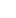in area calculations.

The use of infinitesimals in Leibniz relied upon a heuristic principle called the Law of Continuity
Law of Continuity
The Law of Continuity is a heuristic principle introduced by Leibniz based on earlier work by Nicholas of Cusa and Johannes Kepler. It is the principle that "whatever succeeds for the finite, also succeeds for the infinite"...

: what succeeds for the finite numbers succeeds also for the infinite numbers and vice versa. The 18th century saw routine use of infinitesimals by mathematicians such as Leonhard Euler
Leonhard Euler
Leonhard Euler was a pioneering Swiss mathematician and physicist. He made important discoveries in fields as diverse as infinitesimal calculus and graph theory. He also introduced much of the modern mathematical terminology and notation, particularly for mathematical analysis, such as the notion...

and Joseph Lagrange
Joseph Lagrange
Count Joseph Lagrange was a French soldier who rose through the ranks and gained promotion to the rank of general officer during the French Revolutionary Wars, subsequently pursuing a successful career during the Napoleonic Wars and winning promotion to the top military rank of General of Division....

. Augustin-Louis Cauchy exploited infinitesimals in defining continuity
Continuous function
In mathematics, a continuous function is a function for which, intuitively, "small" changes in the input result in "small" changes in the output. Otherwise, a function is said to be "discontinuous". A continuous function with a continuous inverse function is called "bicontinuous".Continuity of...

and an early form of a Dirac delta function
Dirac delta function
The Dirac delta function, or δ function, is a generalized function depending on a real parameter such that it is zero for all values of the parameter except when the parameter is zero, and its integral over the parameter from −∞ to ∞ is equal to one. It was introduced by theoretical...

. As Cantor and Dedekind were developing more abstact versions of Stevin's continuum, Paul du Bois-Reymond wrote a series of papers on infinitesimal-enriched continua based on growth rates of functions. Du Bois-Reymond's work inspired both Émile Borel
Émile Borel
Félix Édouard Justin Émile Borel was a French mathematician and politician.Borel was born in Saint-Affrique, Aveyron. Along with René-Louis Baire and Henri Lebesgue, he was among the pioneers of measure theory and its application to probability theory. The concept of a Borel set is named in his...

and Thoralf Skolem
Thoralf Skolem
Thoralf Albert Skolem was a Norwegian mathematician known mainly for his work on mathematical logic and set theory.-Life:...

. Skolem developed the first non-standard models of arithmetic in 1934. A mathematical implementation of both the law of continuity and infinitesimals was achieved by Abraham Robinson
Abraham Robinson
Abraham Robinson was a mathematician who is most widely known for development of non-standard analysis, a mathematically rigorous system whereby infinitesimal and infinite numbers were incorporated into mathematics....

in 1961, who developed non-standard analysis
Non-standard analysis
Non-standard analysis is a branch of mathematics that formulates analysis using a rigorous notion of an infinitesimal number.Non-standard analysis was introduced in the early 1960s by the mathematician Abraham Robinson. He wrote:...

based on earlier work by Edwin Hewitt
Edwin Hewitt
Edwin Hewitt was an American mathematician known for his work in abstract harmonic analysis and for his discovery, in collaboration with Leonard Jimmie Savage, of the Hewitt–Savage zero-one law.He received his Ph.D...

in 1948 and Jerzy Łoś in 1955. The hyperreals
Hyperreal number
The system of hyperreal numbers represents a rigorous method of treating the infinite and infinitesimal quantities. The hyperreals, or nonstandard reals, *R, are an extension of the real numbers R that contains numbers greater than anything of the form1 + 1 + \cdots + 1. \, Such a number is...

implement an infinitesimal-enriched continuum and the transfer principle
Transfer principle
In model theory, a transfer principle states that all statements of some language that are true for some structure are true for another structure...

implements Leibniz's law of continuity. The standard part function
Standard part function
In non-standard analysis, the standard part function is a function from the limited hyperreals to the reals, which associates to every hyperreal, the unique real infinitely close to it. As such, it is a mathematical implementation of the historical concept of adequality introduced by Pierre de...

implements Fermat's adequality.

## History of the infinitesimal

The notion of infinitesimally small quantities was discussed by the Eleatic School. Archimedes
Archimedes
Archimedes of Syracuse was a Greek mathematician, physicist, engineer, inventor, and astronomer. Although few details of his life are known, he is regarded as one of the leading scientists in classical antiquity. Among his advances in physics are the foundations of hydrostatics, statics and an...

, in The Method of Mechanical Theorems, was the first to propose a logically rigorous definition of infinitesimals. His Archimedean property
Archimedean property
In abstract algebra and analysis, the Archimedean property, named after the ancient Greek mathematician Archimedes of Syracuse, is a property held by some ordered or normed groups, fields, and other algebraic structures. Roughly speaking, it is the property of having no infinitely large or...

defines a number x as infinite if it satisfies the conditions |x|>1, |x|>1+1, |x|>1+1+1, ..., and infinitesimal if x≠0 and a similar set of conditions holds for 1/x and the reciprocals of the positive integers. A number system is said to be Archimedean if it contains no infinite or infinitesimal members. In the ancient Greek system of mathematics, 1 represents the length of some line segment which has arbitrarily been picked as the unit of measurement.

The Indian mathematician
Indian mathematics
Indian mathematics emerged in the Indian subcontinent from 1200 BCE until the end of the 18th century. In the classical period of Indian mathematics , important contributions were made by scholars like Aryabhata, Brahmagupta, and Bhaskara II. The decimal number system in use today was first...

Bhāskara II (1114–1185) described a geometric technique for expressing the change in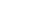in terms of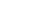times a change in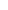. The Persian mathematician Sharaf al-Dīn al-Tūsī (1135–1213) used ancient and medieval methods to study the number of roots of cubic polynomials. The Kerala school of astronomy and mathematics in the 14th to 16th centuries made use of an intuitive notion of a limit
Limit of a sequence
The limit of a sequence is, intuitively, the unique number or point L such that the terms of the sequence become arbitrarily close to L for "large" values of n...

to compute several series
Series (mathematics)
A series is the sum of the terms of a sequence. Finite sequences and series have defined first and last terms, whereas infinite sequences and series continue indefinitely....

expansions.

A key notion of adequality
Adequality
In the history of infinitesimal calculus, adequality is a technique developed by Pierre de Fermat. Fermat said he borrowed the term from Diophantus. Adequality was a technique first used to find maxima for functions and then adapted to find tangent lines to curves...

was already present in the work of Pierre de Fermat
Pierre de Fermat
Pierre de Fermat was a French lawyer at the Parlement of Toulouse, France, and an amateur mathematician who is given credit for early developments that led to infinitesimal calculus, including his adequality...

prior to the invention of the calculus. When Newton
Isaac Newton
Sir Isaac Newton PRS was an English physicist, mathematician, astronomer, natural philosopher, alchemist, and theologian, who has been "considered by many to be the greatest and most influential scientist who ever lived."...

and Leibniz
Gottfried Leibniz
Gottfried Wilhelm Leibniz was a German philosopher and mathematician. He wrote in different languages, primarily in Latin , French and German ....

invented the calculus
Infinitesimal calculus
Infinitesimal calculus is the part of mathematics concerned with finding slope of curves, areas under curves, minima and maxima, and other geometric and analytic problems. It was independently developed by Gottfried Leibniz and Isaac Newton starting in the 1660s...

, they made use of infinitesimals. The use of infinitesimals was attacked as incorrect by Bishop Berkeley
George Berkeley
George Berkeley , also known as Bishop Berkeley , was an Irish philosopher whose primary achievement was the advancement of a theory he called "immaterialism"...

in his work The Analyst
The Analyst
The Analyst, subtitled "A DISCOURSE Addressed to an Infidel MATHEMATICIAN. WHEREIN It is examined whether the Object, Principles, and Inferences of the modern Analysis are more distinctly conceived, or more evidently deduced, than Religious Mysteries and Points of Faith", is a book published by...

. Mathematicians, scientists, and engineers continued to use infinitesimals to produce correct results. In the second half of the nineteenth century, the calculus was reformulated by Karl Weierstrass
Karl Weierstrass
Karl Theodor Wilhelm Weierstrass was a German mathematician who is often cited as the "father of modern analysis".- Biography :Weierstrass was born in Ostenfelde, part of Ennigerloh, Province of Westphalia....

, Cantor
Georg Cantor
Georg Ferdinand Ludwig Philipp Cantor was a German mathematician, best known as the inventor of set theory, which has become a fundamental theory in mathematics. Cantor established the importance of one-to-one correspondence between the members of two sets, defined infinite and well-ordered sets,...

, Dedekind
Dedekind
-People:* Brendon Dedekind , South African swimmer* Friedrich Dedekind , German humanist, theologian, and bookseller* Richard Dedekind , German mathematician...

, and others using the (ε, δ)-definition of limit and set theory
Set theory
Set theory is the branch of mathematics that studies sets, which are collections of objects. Although any type of object can be collected into a set, set theory is applied most often to objects that are relevant to mathematics...

. While infinitesimals eventually disappeared from the calculus, their mathematical study continued through the work of Levi-Civita
Tullio Levi-Civita
Tullio Levi-Civita, FRS was an Italian mathematician, most famous for his work on absolute differential calculus and its applications to the theory of relativity, but who also made significant contributions in other areas. He was a pupil of Gregorio Ricci-Curbastro, the inventor of tensor calculus...

and others, throughout the late nineteenth and the twentieth centuries, as documented by Ehrlich (2006). In the 20th century, it was found that infinitesimals could serve as a basis for calculus and analysis.

## First-order properties

In extending the real numbers to include infinite and infinitesimal quantities, one typically wishes to be as conservative as possible by not changing any of their elementary properties. This guarantees that as many familiar results as possible will still be available. Typically elementary means that there is no quantification over sets, but only over elements. This limitation allows statements of the form "for any number x..." For example, the axiom that states "for any number x, x + 0 = x" would still apply. The same is true for quantification over several numbers, e.g., "for any numbers x and y, xy = yx." However, statements of the form "for any set S of numbers ..." may not carry over. This limitation on quantification is referred to as first-order logic
First-order logic
First-order logic is a formal logical system used in mathematics, philosophy, linguistics, and computer science. It goes by many names, including: first-order predicate calculus, the lower predicate calculus, quantification theory, and predicate logic...

.

It is clear that the resulting extended number system cannot agree with the reals on all properties that can be expressed by quantification over sets, because the goal is to construct a nonarchimedean system, and the Archimedean principle can be expressed by quantification over sets. Similarly, the completeness property cannot be expected to carry over, because the reals are the unique complete ordered field (up to isomorphism).

We can distinguish three levels at which a nonarchimedean number system could have first-order properties compatible with those of the reals:
1. An ordered field
Ordered field
In mathematics, an ordered field is a field together with a total ordering of its elements that is compatible with the field operations. Historically, the axiomatization of an ordered field was abstracted gradually from the real numbers, by mathematicians including David Hilbert, Otto Hölder and...

obeys all the usual axioms of the real number system that can be stated in first-order logic. For example, the commutativity
Commutativity
In mathematics an operation is commutative if changing the order of the operands does not change the end result. It is a fundamental property of many binary operations, and many mathematical proofs depend on it...

axiom x + y = y + x holds.
2. A real closed field
Real closed field
In mathematics, a real closed field is a field F that has the same first-order properties as the field of real numbers. Some examples are the field of real numbers, the field of real algebraic numbers, and the field of hyperreal numbers.-Definitions:...

has all the first-order properties of the real number system, regardless of whether they are usually taken as axiomatic, for statements involving the basic ordered-field relations +, * , and ≤. This is a stronger condition than obeying the ordered-field axioms. More specifically, one includes additional first-order properties, such as the existence of a root for every odd-degree polynomial. For example, every number must have a cube root.
3. The system could have all the first-order properties of the real number system for statements involving any relations (regardless of whether those relations can be expressed using +, *, and ≤). For example, there would have to be a sine
Sine
In mathematics, the sine function is a function of an angle. In a right triangle, sine gives the ratio of the length of the side opposite to an angle to the length of the hypotenuse.Sine is usually listed first amongst the trigonometric functions....

function that is well defined for infinite inputs; the same is true for every real function.

Systems in category 1, at the weak end of the spectrum, are relatively easy to construct, but do not allow a full treatment of classical analysis using infinitesimals in the spirit of Newton and Leibniz. For example, the transcendental functions are defined in terms of infinite limiting processes, and therefore there is typically no way to define them in first-order logic. Increasing the analytic strength of the system by passing to categories 2 and 3, we find that the flavor of the treatment tends to become less constructive, and it becomes more difficult to say anything concrete about the hierarchical structure of infinities and infinitesimals.

#### Laurent series

An example from category 1 above is the field of Laurent series
Laurent series
In mathematics, the Laurent series of a complex function f is a representation of that function as a power series which includes terms of negative degree. It may be used to express complex functions in cases where...

with a finite number of negative-power terms. For example, the Laurent series consisting only of the constant term 1 is identified with the real number 1, and the series with only the linear term x is thought of as the simplest infinitesimal, from which the other infinitesimals are constructed. Dictionary ordering is used, which is equivalent to considering higher powers of x as negligible compared to lower powers. David O. Tall
David O. Tall
David Orme Tall is a mathematics education theorist at the University of Warwick. One of his most influential works is the joint paper with Vinner Concept image and concept definition.... The "concept image" is a notion in cognitive theory. It consists of all the cognitive structure in the...

refers to this system as the super-reals, not to be confused with the superreal number
Superreal number
In abstract algebra, the superreal numbers are a class of extensions of the real numbers, introduced by H. Garth Dales and W. Hugh Woodin as a generalization of the hyperreal numbers and primarily of interest in non-standard analysis, model theory, and the study of Banach algebras...

system of Dales and Woodin. Since a Taylor series evaluated with a Laurent series as its argument is still a Laurent series, the system can be used to do calculus on transcendental functions if they are analytic. These infinitesimals have different first-order properties than the reals because, for example, the basic infinitesimal x does not have a square root.

#### The Levi-Civita field

The Levi-Civita field
Levi-Civita field
In mathematics, the Levi-Civita field, named after Tullio Levi-Civita, is a non-Archimedean ordered field, i.e., a system of numbers containing infinite and infinitesimal quantities...

is similar to the Laurent series, but is algebraically closed. For example, the basic infinitesimal x has a square root. This field is rich enough to allow a significant amount of analysis to be done, but its elements can still be represented on a computer in the same sense that real numbers can be represented in floating point. It has applications to numerical differentiation in cases that are intractable by symbolic differentiation or finite-difference methods.

#### Transseries

The field of transseries is larger than the Levi-Civita field. An example of a transseries is: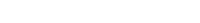where for purposes of ordering x is considered to be infinite.

### Surreal numbers

Conway's surreal numbers fall into category 2. They are a system that was designed to be as rich as possible in different sizes of numbers, but not necessarily for convenience in doing analysis. Certain transcendental functions can be carried over to the surreals, including logarithms and exponenentials, but most, e.g., the sine function, cannot. The existence of any particular surreal number, even one that has a direct counterpart in the reals, is not known a priori, and must be proved.

### The hyperreal system

The most widespread technique for handling infinitesimals is the hyperreals, developed by Abraham Robinson
Abraham Robinson
Abraham Robinson was a mathematician who is most widely known for development of non-standard analysis, a mathematically rigorous system whereby infinitesimal and infinite numbers were incorporated into mathematics....

in the 1960s. They fall into category 3 above, having been designed that way in order to allow all of classical analysis to be carried over from the reals. This property of being able to carry over all relations in a natural way is known as the transfer principle
Transfer principle
In model theory, a transfer principle states that all statements of some language that are true for some structure are true for another structure...

, proved by Jerzy Łoś in 1955. For example, the transcendental function sin has a natural counterpart *sin that takes a hyperreal input and gives a hyperreal output, and similarly the set of natural numbers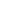has a natural counterpart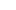, which contains both finite and infinite integers. A proposition such as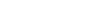carries over to the hyperreals as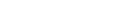.

### Superreals

The superreal number
Superreal number
In abstract algebra, the superreal numbers are a class of extensions of the real numbers, introduced by H. Garth Dales and W. Hugh Woodin as a generalization of the hyperreal numbers and primarily of interest in non-standard analysis, model theory, and the study of Banach algebras...

system of Dales and Woodin is a generalization of the hyperreals. It is different from the super-real system defined by David Tall
David O. Tall
David Orme Tall is a mathematics education theorist at the University of Warwick. One of his most influential works is the joint paper with Vinner Concept image and concept definition.... The "concept image" is a notion in cognitive theory. It consists of all the cognitive structure in the...

.

### Smooth infinitesimal analysis

Synthetic differential geometry
Synthetic differential geometry
In mathematics, synthetic differential geometry is a reformulation of differential geometry in the language of topos theory, in the context of an intuitionistic logic characterized by a rejection of the law of excluded middle. There are several insights that allow for such a reformulation...

or smooth infinitesimal analysis
Smooth infinitesimal analysis
Smooth infinitesimal analysis is a mathematically rigorous reformulation of the calculus in terms of infinitesimals. Based on the ideas of F. W. Lawvere and employing the methods of category theory, it views all functions as being continuous and incapable of being expressed in terms of discrete...

have roots in category theory
Category theory
Category theory is an area of study in mathematics that examines in an abstract way the properties of particular mathematical concepts, by formalising them as collections of objects and arrows , where these collections satisfy certain basic conditions...

. This approach departs from the classical logic used in conventional mathematics by denying the general applicability of the law of excluded middle
Law of excluded middle
In logic, the law of excluded middle is the third of the so-called three classic laws of thought. It states that for any proposition, either that proposition is true, or its negation is....

— i.e., not (ab) does not have to mean a = b. A nilsquare or nilpotent
Nilpotent
In mathematics, an element x of a ring R is called nilpotent if there exists some positive integer n such that xn = 0....

infinitesimal can then be defined. This is a number x where x2 = 0 is true, but x = 0 need not be true at the same time. Since the background logic is intuitionistic logic
Intuitionistic logic
Intuitionistic logic, or constructive logic, is a symbolic logic system differing from classical logic in its definition of the meaning of a statement being true. In classical logic, all well-formed statements are assumed to be either true or false, even if we do not have a proof of either...

, it is not immediately clear how to classify this system with regard to classes 1, 2, and 3. Intuitionistic analogues of these classes would have to be developed first.

## Infinitesimal delta functions

Cauchy used an infinitesimalto write down a unit impulse, infinitely tall and narrow Dirac-type delta function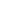satisfying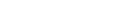in a number of articles in 1827, see Laugwitz (1989). Cauchy defined an infinitesimal in 1821 (Cours d'Analyse) in terms of a sequence tending to zero. Namely, such a null sequence becomes an infinitesimal in Cauchy's and Lazare Carnot
Lazare Carnot
Lazare Nicolas Marguerite, Comte Carnot , the Organizer of Victory in the French Revolutionary Wars, was a French politician, engineer, and mathematician.-Education and early life:...

's terminology.

Modern set-theoretic approaches allow one to define infinitesimals via the ultrapower construction, where a null sequence becomes an infinitesimal in the sense of an equivalence class modulo a relation defined in terms of a suitable ultrafilter
Ultrafilter
In the mathematical field of set theory, an ultrafilter on a set X is a collection of subsets of X that is a filter, that cannot be enlarged . An ultrafilter may be considered as a finitely additive measure. Then every subset of X is either considered "almost everything" or "almost nothing"...

. The article by Yamashita (2007) contains a bibliography on modern Dirac delta function
Dirac delta function
The Dirac delta function, or δ function, is a generalized function depending on a real parameter such that it is zero for all values of the parameter except when the parameter is zero, and its integral over the parameter from −∞ to ∞ is equal to one. It was introduced by theoretical...

s in the context of an infinitesimal-enriched continuum provided by the hyperreals
Hyperreal number
The system of hyperreal numbers represents a rigorous method of treating the infinite and infinitesimal quantities. The hyperreals, or nonstandard reals, *R, are an extension of the real numbers R that contains numbers greater than anything of the form1 + 1 + \cdots + 1. \, Such a number is...

.

## Logical properties

The method of constructing infinitesimals of the kind used in nonstandard analysis depends on the model
Model theory
In mathematics, model theory is the study of mathematical structures using tools from mathematical logic....

and which collection of axiom
Axiom
In traditional logic, an axiom or postulate is a proposition that is not proven or demonstrated but considered either to be self-evident or to define and delimit the realm of analysis. In other words, an axiom is a logical statement that is assumed to be true...

s are used. We consider here systems where infinitesimals can be shown to exist.

In 1936 Maltsev
Maltsev
Maltsev or Maltseva is a Russian surname which may refer to:-People:* Anatoly Maltsev, a mathematician* Aleksandr Maltsev, an ice hockey player.* Yuri Maltsev, economist and writer...

proved the compactness theorem
Compactness theorem
In mathematical logic, the compactness theorem states that a set of first-order sentences has a model if and only if every finite subset of it has a model...

. This theorem is fundamental for the existence of infinitesimals as it proves that it is possible to formalise them. A consequence of this theorem is that if there is a number system in which it is true that for any positive integer n there is a positive number x such that 0 < x < 1/n, then there exists an extension of that number system in which it is true that there exists a positive number x such that for any positive integer n we have 0 < x < 1/n. The possibility to switch "for any" and "there exists" is crucial. The first statement is true in the real numbers as given in ZFC set theory
Set theory
Set theory is the branch of mathematics that studies sets, which are collections of objects. Although any type of object can be collected into a set, set theory is applied most often to objects that are relevant to mathematics...

: for any positive integer n it is possible to find a real number between 1/n and zero, but this real number will depend on n. Here, one chooses n first, then one finds the corresponding x. In the second expression, the statement says that there is an x (at least one), chosen first, which is between 0 and 1/n for any n. In this case x is infinitesimal. This is not true in the real numbers (R) given by ZFC. Nonetheless, the theorem proves that there is a model (a number system) in which this will be true. The question is: what is this model? What are its properties? Is there only one such model?

There are in fact many ways to construct such a one-dimensional
Dimension
In physics and mathematics, the dimension of a space or object is informally defined as the minimum number of coordinates needed to specify any point within it. Thus a line has a dimension of one because only one coordinate is needed to specify a point on it...

linearly ordered set of numbers, but fundamentally, there are two different approaches:
1) Extend the number system so that it contains more numbers than the real numbers.

2) Extend the axioms (or extend the language) so that the distinction between the infinitesimals and non-infinitesimals can be made in the real numbers themselves.

In 1960, Abraham Robinson
Abraham Robinson
Abraham Robinson was a mathematician who is most widely known for development of non-standard analysis, a mathematically rigorous system whereby infinitesimal and infinite numbers were incorporated into mathematics....

provided an answer following the first approach. The extended set is called the hyperreal
Hyperreal number
The system of hyperreal numbers represents a rigorous method of treating the infinite and infinitesimal quantities. The hyperreals, or nonstandard reals, *R, are an extension of the real numbers R that contains numbers greater than anything of the form1 + 1 + \cdots + 1. \, Such a number is...

s and contains numbers less in absolute value than any positive real number. The method may be considered relatively complex but it does prove that infinitesimals exist in the universe of ZFC set theory. The real numbers are called standard numbers and the new non-real hyperreals are called nonstandard.

In 1977 Edward Nelson
Edward Nelson
Edward Nelson is a professor in the Mathematics Department at Princeton University. He is known for his work on mathematical physics and mathematical logic...

provided an answer following the second approach. The extended axioms are IST, which stands either for Internal Set Theory
Internal set theory
Internal set theory is a mathematical theory of sets developed by Edward Nelson that provides an axiomatic basis for a portion of the non-standard analysis introduced by Abraham Robinson. Instead of adding new elements to the real numbers, the axioms introduce a new term, "standard", which can be...

or for the initials of the three extra axioms: Idealization, Standardization, Transfer. In this system we consider that the language is extended in such a way that we can express facts about infinitesimals. The real numbers are either standard or nonstandard. An infinitesimal is a nonstandard real number which is less, in absolute value, than any positive standard real number.

In 2006 Karel Hrbacek developed an extension of Nelson's approach in which the real numbers are stratified in (infinitely) many levels i.e., in the coarsest level there are no infinitesimals nor unlimited numbers. Infinitesimals are in a finer level and there are also infinitesimals with respect to this new level and so on.
The source of this article is wikipedia, the free encyclopedia.  The text of this article is licensed under the GFDL.